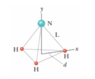# Center of mass in NH3

• JessicaHelena
In summary, the x coordinate of the molecule's center of mass is 9.40 ✕ 10^−11 m and the y coordinate of the molecule's center of mass is 10.14 ✕ 10^−11 m.

## Homework Statement

In the ammonia (NH3) molecule of the figure, the three hydrogen (H) atoms form an equilateral triangle, with the center of the triangle at distance d = 9.40 ✕ 10^−11 m from each hydrogen atom. The nitrogen (N) atom is at the apex of a pyramid, with the three hydrogen atoms forming the base. The nitrogen-to-hydrogen atomic mass ratio is 13.9, and the nitrogen-to-hydrogen distance is L = 10.14 ✕ 10^−11 m.

(a) What is the x coordinate of the molecule's center of mass?

(b) What is the y coordinate of the molecule's center of mass?

## Homework Equations

x_cm = sum of (m*x)/M
(same for y)

## The Attempt at a Solution

(a) I'm not quite sure how to deal with the two hydrogens towards the negative x-axis — they're not exactly on the axis at all.

(b) None of the H's have any y values, so I did 13.9*sqrt(L^2-d^2)/(13.9+3*1) where 3*1 accounted for the masses of the three H's, but apparently that answer is wrong, and I've no idea why it is. (I got 1.137*10^-(10).)

#### Attachments

•Screen Shot 2018-09-24 at 2.18.23 AM.png
4.1 KB · Views: 1,236
You need to work out the geometry better for part (a). This is really just a geometry problem, with an equilateral triangle, and the origin (x=0) is at the centroid of that equilateral triangle. This can be readily shown to be the x-center of mass, and we can show that once we have the coordinates. ## \\ ## (Edit: Go to the bottom, and read that part first. Looking over what you did, you have already done most of what the problem asks for). ## \\ ## (They really should call their y-direction z, and the plane of the 3 hydrogen atoms should be the x-y plane, but I think they were trying to make it overly simple). I would recommend you make this change, and compute the location of the coordinates of the vertices of an equilateral triangle in the x-y plane, when the centroid is at ##(x,y) ## of ## (d,0 ) ##, and compute ## (x,y) ## for the other two vertices. Then we can show with a little arithmetic that the center of mass in the x-y plane is at (0,0). Otherwise, we can simply assume that the x-center of mass is ## x=0 ## as defined in this problem, but that makes it almost too easy.## \\ ## ## \\ ## The location of the center of mass in the ## y ## direction is very readily computed. They give you the hypotenuse distance, but you need to compute the distance ## d ## from the centroid of the equilateral triangle to the hydrogen atom(s). (Edit: They give you that distance ## d ##. That makes it very easy). Once you have that, it is a simple matter using the Pythagorean theorem to compute the y-height of the nitrogen atom. Computing the y-center of mass then follows with a very simple equation. (Edit: And I see you did that. Very good ).## \\ ## Edit: Looking over your work for part (b), it looks correct. Check your arithmetic=I get a very different answer.

Last edited:
for the x_cm, I too thought that it would be 0, but the answer is 9.40 ✕ 10^−11 m... Do you have any idea why this could be?

JessicaHelena said:
•